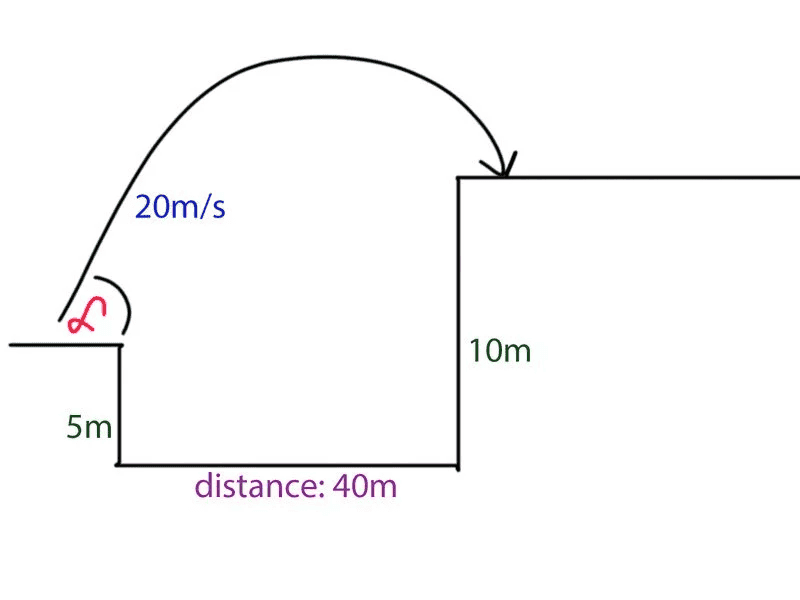# Projectile launch angle

• dejavu333

#### dejavu333

Homework Statement
velocity:20m/s we're on 5m, target is on 10m. What are the possible launching angles? The diatance is 40m
Air resistance can be neglected.
Relevant Equations
none
if the target is lower than me, the formula is below, but if it's higher as in the task, a whole different formula is required I think.
a = 9.8 * distance^2 / velocity^2
phi = Arctan(distance / height_diff)
angle_if_elevation = ( Arccos((a - height_diff) / Sqroot(height_diff^2) + distance^2)) + phi ) / 2

Your left out an open paren, so:
angle_if_elevation = ( Arccos((a - height_diff) / Sqroot((height_diff^2) + distance^2)) + phi ) / 2

The same formulas are used - with the "height_diff" being negative.
But, you may not get results to your expectation.
My guess is that your arccos computation is giving you a problem.

What do you think is the source of that problem?

I think this is your graphic:Last edited: